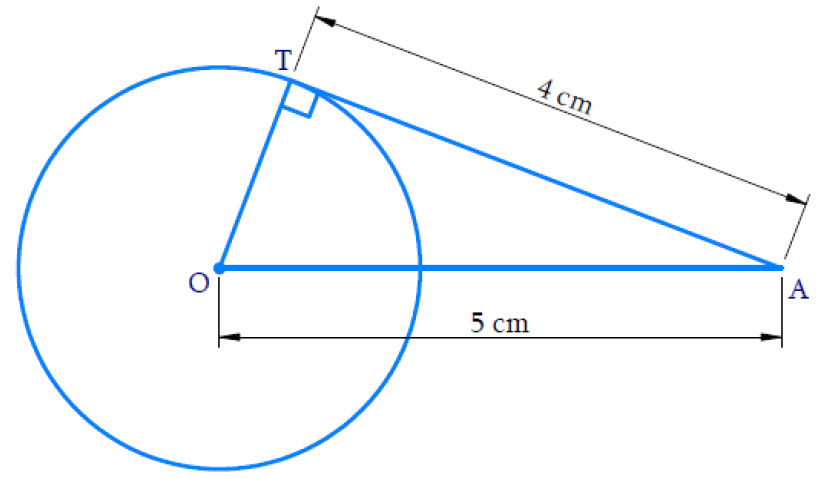# Ex.10.2 Q6 Circles Solution - NCERT Maths Class 10

Go back to  'Ex.10.2'

## Question

The length of a tangent from a point $$A$$ at distance $$\text{5 cm}$$ from the center of the circle is $$\text{4 cm.}$$ Find the radius of the circle.

Video Solution
Circles
Ex 10.2 | Question 6

## Text Solution

What is Known?

Length of tangent from point $$A = \text{4 cm.}$$

Distance between the centre of the circle and point $$A$$ is $$\text{5 cm.}$$

What is Unknown?

Reasoning:Tangent at any point of a circle is perpendicular to the radius through the point of contact.

Therefore, $$\angle {OTA} = {90^ \circ }$$ and $$\Delta {OTA}$$ is right angled triangle.

Steps:

By Pythagoras theorem

\begin{align} {O A} ^ { 2 } &= {O T} ^ { 2 } + {A T} ^ { 2 } & \\ 5 ^ { 2 } & = {O T} ^ { 2 } + 4 ^ { 2 } \\ {O T} ^ { 2 } & = 25 - 16 \\ {O T} ^ { 2 } & = 9 \\ {O T} & = \pm 3 \end{align}

Radius $$OT$$ cannot be negative. Hence, the radius is $$\text{3 cm.}$$

Learn from the best math teachers and top your exams

• Live one on one classroom and doubt clearing
• Practice worksheets in and after class for conceptual clarity
• Personalized curriculum to keep up with school